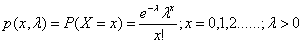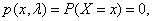# Statistics Assignment Help With Poisson Distribution

## 5.3 Poisson Distribution:

Poisson distribution was discovered by the French mathematician and physicist Simeon Denis Poisson who published it in 1837. Poisson distribution is a limiting case of the binomial distribution under the following conditions.(i) n, the number of trials is indefinitely large, i.e., nà ∞.

(ii) p, the constant probability of success for each trial is indefinitely small, i.e., pà 0.

(iii) np = λ, is finite. Thus p = λ/n, q = 1-λ/n, where λ is a positive real number.

A random variable X is said to follow a Poisson distribution if it assumes only non-negative values and its probability mass function is given byotherwise

Here λ is known as the parameter of the distribution.

We shall use the notation X~ P(λ) to denote that X is a Poisson variate with parameter λ.